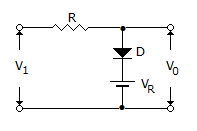# Electronics and Communication Engineering - Analog Electronics

16.

The enhancement type MOSFET is called a

 A. normally on MOSFET B. normally off MOSFET C. P-type MOSFET D. N-type MOSFET

Explanation:

No answer description available for this question. Let us discuss.

17.

The input gate current of a FET is

 A. a few amperes B. a few milli amperes C. a few micro amperes D. negligible

Explanation:

No answer description available for this question. Let us discuss.

18.

For maximum unclipped output from a CE amplifier, the Q point should be

 A. at the centre of dc load line B. at the centre of ac load line C. at the centre of collector current D. none of the above

Explanation:

No answer description available for this question. Let us discuss.

19.

The circuit shown in figure will act asA. differentiator B. adder C. clipper D. clamper

Explanation:

No answer description available for this question. Let us discuss.

20.

Which of the following best represents the input impedance of an actual op-amp?

 A. 10 ohm B. 1 k ohm C. 1 M ohm D. 100 M ohm